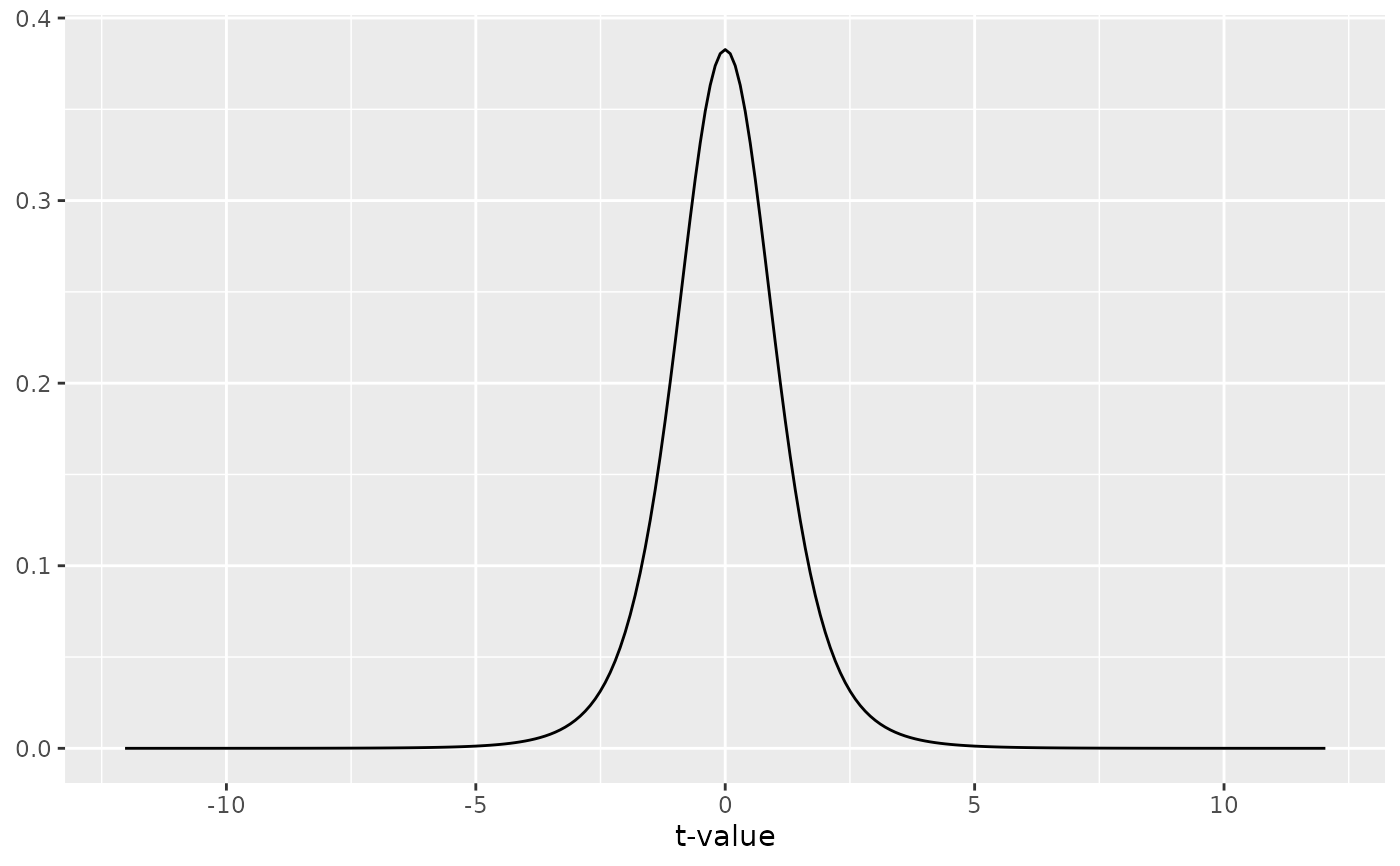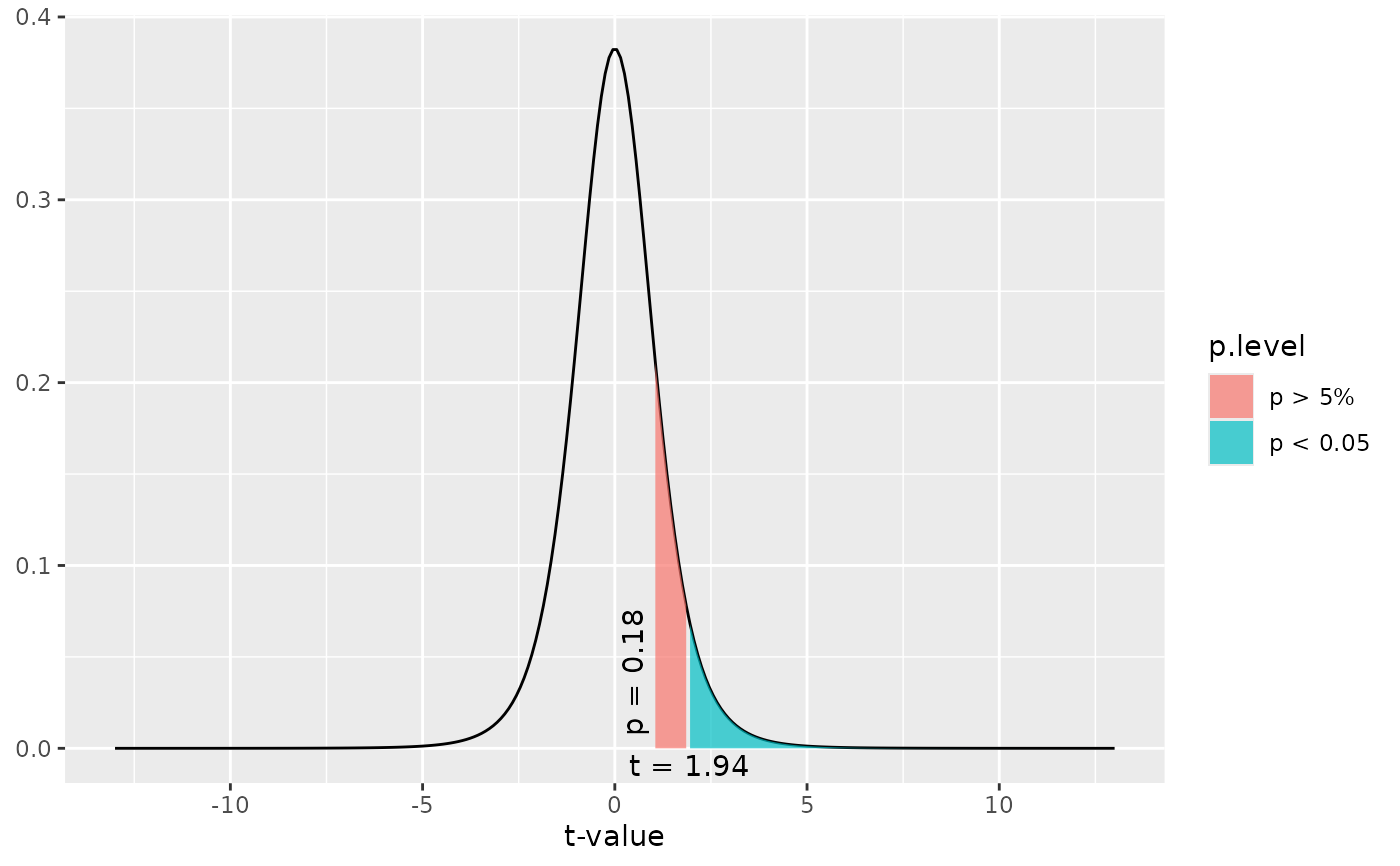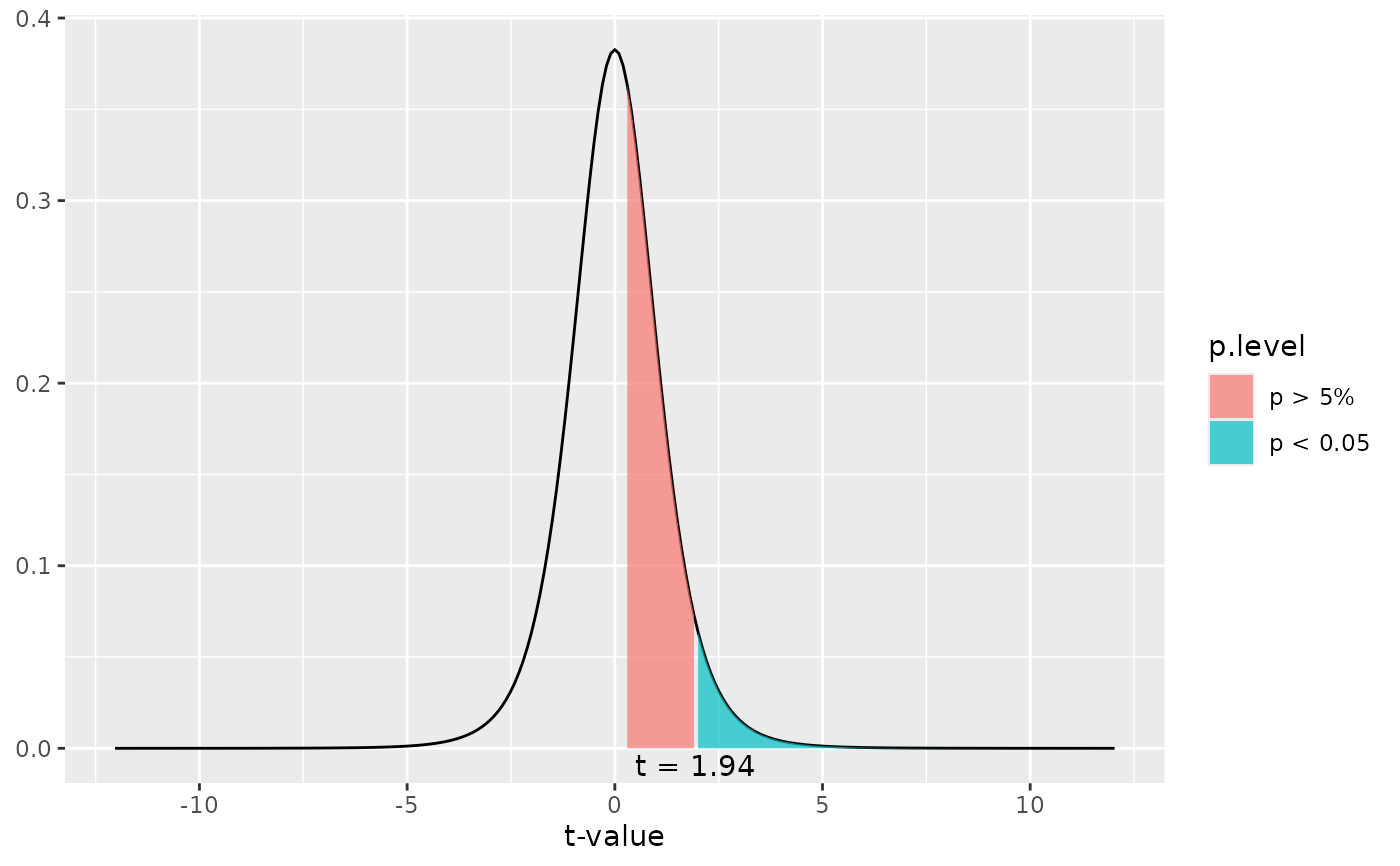This function plots a simple t-distribution or a t-distribution with shaded areas that indicate at which t-value a significant p-level is reached.

dist_t(
t = NULL,
deg.f = NULL,
p = NULL,
xmax = NULL,
geom.colors = NULL,
geom.alpha = 0.7
)

Arguments

t

Numeric, optional. If specified, a t-distribution with deg.f degrees of freedom is plotted and a shaded area at t value position is plotted that indicates whether or not the specified value is significant or not. If both t and p are not specified, a distribution without shaded area is plotted.

deg.f

Numeric. The degrees of freedom for the t-distribution. Needs to be specified.

p

Numeric, optional. If specified, a t-distribution with deg.f degrees of freedom is plotted and a shaded area at the position where the specified p-level starts is plotted. If both t and p are not specified, a distribution without shaded area is plotted.

xmax

Numeric, optional. Specifies the maximum x-axis-value. If not specified, the x-axis ranges to a value where a p-level of 0.00001 is reached.

geom.colors

user defined color for geoms. See 'Details' in plot_grpfrq.

geom.alpha

Specifies the alpha-level of the shaded area. Default is 0.7, range between 0 to 1.

Examples

# a simple t-distribution
# for 6 degrees of freedom
dist_t(deg.f = 6)# a t-distribution for 6 degrees of freedom,
# and a shaded area starting at t-value of one.
# With a df of 6, a t-value of 1.94 would be "significant".
dist_t(t = 1, deg.f = 6)# a t-distribution for 6 degrees of freedom,
# and a shaded area starting at p-level of 0.4